# 一、list

Python内置的一种数据类型是列表：list。list是一种有序的集合，可以随时添加和删除其中的元素。

``````classmates = ['Michael', 'Bob', 'Tracy']
print(classmates)``````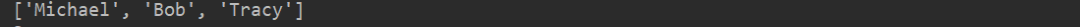# 1. 获得list元素的个数：

``````classmates = ['Michael', 'Bob', 'Tracy']
print(len(classmates))``````

``````classmates = ['Michael', 'Bob', 'Tracy']

print(classmates)

print(classmates)

print(classmates)

print(classmates)``````

``print(classmates[-1])``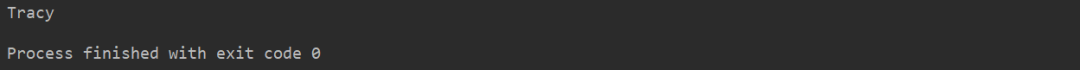``````classmates = ['Michael', 'Bob', 'Tracy']

print(classmates[-1])

print(classmates[-2])

print(classmates[-3])

print(classmates[-4])``````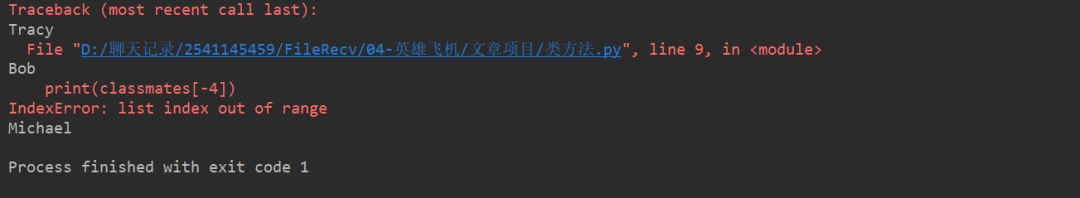# 2. list是一个可变的有序表，往list中追加元素到末尾：

``````classmates = ['Michael', 'Bob', 'Tracy']

print(classmates)``````

``````classmates = ['Michael', 'Bob', 'Tracy']
#替换
classmates.insert(1, 'Jack')

print(classmates)``````# 1. 删除list末尾的元素

``````classmates = ['Michael', 'Bob', 'Tracy']

print(classmates.pop())

print( classmates)
['Michael', 'Jack', 'Bob', 'Tracy']``````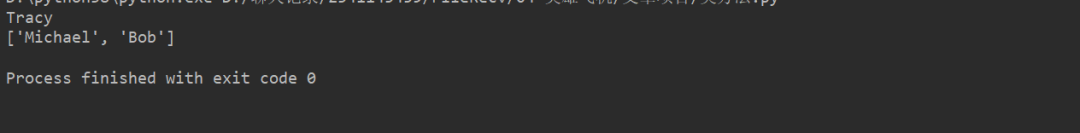# 2. 删除指定位置的元素，用pop(i)方法，其中i是索引位置。

``````classmates.pop(1)

print(classmates)``````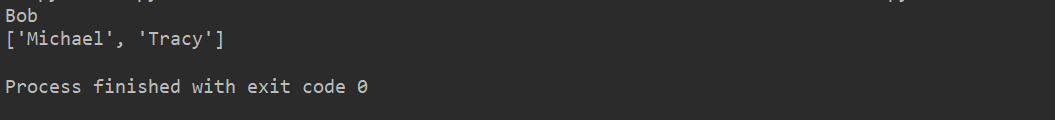# 3. 把某个元素替换成别的元素，可以直接赋值给对应的索引位置：

``````classmates = ['Michael', 'Bob', 'Tracy']

classmates = 'Sarah'

print(classmates)``````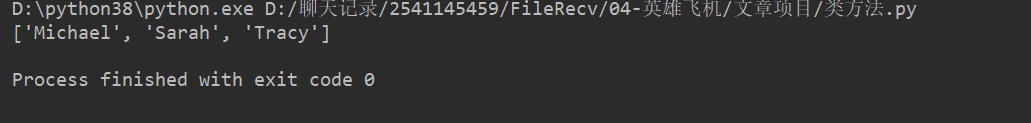list里面的元素的数据类型也可以不同，比如：

``L = ['Apple', 123, True]``

list元素也可以是另一个list，比如：

``````s = ['python', 'java', ['asp', 'php'], 'scheme']
print(len(s))``````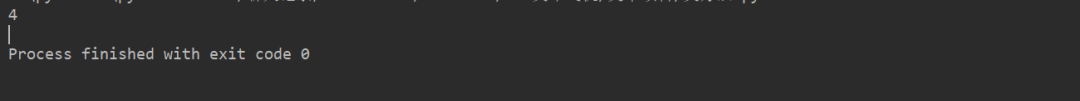``````p = ['asp', 'php']
s = ['python', 'java', p, 'scheme']``````

``````L = []
len(L)``````

# 二、总结

IT共享之家

****看完本文有收获？请转发分享给更多的人****—————— End ——————